# Prim's algorithm

﻿
Prim's algorithm

In computer science, Prim's algorithm is a greedy algorithm that finds a minimum spanning tree for a connected weighted undirected graph. This means it finds a subset of the edges that forms a tree that includes every vertex, where the total weight of all the edges in the tree is minimized. The algorithm was developed in 1930 by Czech mathematician Vojtěch Jarník and later independently by computer scientist Robert C. Prim in 1957 and rediscovered by Edsger Dijkstra in 1959. Therefore it is also sometimes called the DJP algorithm, the Jarník algorithm, or the Prim–Jarník algorithm.

Other algorithms for this problem include Kruskal's algorithm and Borůvka's algorithm.

## DescriptionPrim's algorithm has many applications, such as in the generation of this maze, which applies Prim's algorithm to a randomly weighted grid graph.

The only spanning tree of the empty graph (with an empty vertex set) is again the empty graph. The following description assumes that this special case is handled separately.

The algorithm continuously increases the size of a tree, one edge at a time, starting with a tree consisting of a single vertex, until it spans all vertices.

• Input: A non-empty connected weighted graph with vertices V and edges E (the weights can be negative).
• Initialize: Vnew = {x}, where x is an arbitrary node (starting point) from V, Enew = {}
• Repeat until Vnew = V:
• Choose an edge (u, v) with minimal weight such that u is in Vnew and v is not (if there are multiple edges with the same weight, any of them may be picked)
• Add v to Vnew, and (u, v) to Enew
• Output: Vnew and Enew describe a minimal spanning tree

## Time complexity

Minimum edge weight data structure Time complexity (total)
binary heap and adjacency list O((V + E) log V) = O(E log V)
Fibonacci heap and adjacency list O(E + V log V)

A simple implementation using an adjacency matrix graph representation and searching an array of weights to find the minimum weight edge to add requires O(V2) running time. Using a simple binary heap data structure and an adjacency list representation, Prim's algorithm can be shown to run in time O(E log V) where E is the number of edges and V is the number of vertices. Using a more sophisticated Fibonacci heap, this can be brought down to O(E + V log V), which is asymptotically faster when the graph is dense enough that E is Ω(V).

## Example run

Image DescriptionThis is our original weighted graph. The numbers near the edges indicate their weight.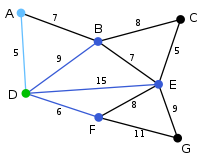Vertex D has been arbitrarily chosen as a starting point. Vertices A, B, E and F are connected to D through a single edge. A is the vertex nearest to D and will be chosen as the second vertex along with the edge AD.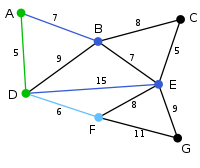The next vertex chosen is the vertex nearest to either D or A. B is 9 away from D and 7 away from A, E is 15, and F is 6. F is the smallest distance away, so we highlight the vertex F and the arc DF.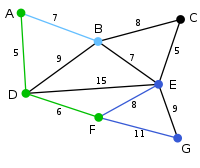The algorithm carries on as above. Vertex B, which is 7 away from A, is highlighted.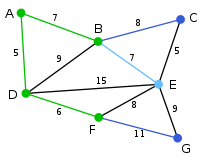In this case, we can choose between C, E, and G. C is 8 away from B, E is 7 away from B, and G is 11 away from F. E is nearest, so we highlight the vertex E and the arc BE.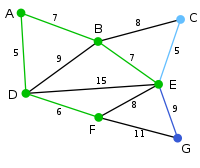Here, the only vertices available are C and G. C is 5 away from E, and G is 9 away from E. C is chosen, so it is highlighted along with the arc EC.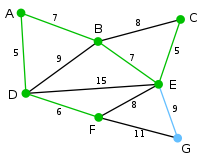Vertex G is the only remaining vertex. It is 11 away from F, and 9 away from E. E is nearer, so we highlight it and the arc EG.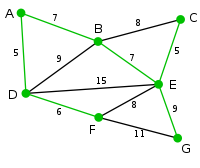Now all the vertices have been selected and the minimum spanning tree is shown in green. In this case, it has weight 39.
U Edge(u,v) V \ U
{} {A,B,C,D,E,F,G}
{D} (D,A) = 5 V
(D,B) = 9
(D,E) = 15
(D,F) = 6
{A,B,C,E,F,G}
{A,D} (D,B) = 9
(D,E) = 15
(D,F) = 6 V
(A,B) = 7
{B,C,E,F,G}
{A,D,F} (D,B) = 9
(D,E) = 15
(A,B) = 7 V
(F,E) = 8
(F,G) = 11
{B,C,E,G}
{A,B,D,F} (B,C) = 8
(B,E) = 7 V
(D,B) = 9 cycle
(D,E) = 15
(F,E) = 8
(F,G) = 11
{C,E,G}
{A,B,D,E,F} (B,C) = 8
(D,B) = 9 cycle
(D,E) = 15 cycle
(E,C) = 5 V
(E,G) = 9
(F,E) = 8 cycle
(F,G) = 11
{C,G}
{A,B,C,D,E,F} (B,C) = 8 cycle
(D,B) = 9 cycle
(D,E) = 15 cycle
(E,G) = 9 V
(F,E) = 8 cycle
(F,G) = 11
{G}
{A,B,C,D,E,F,G} (B,C) = 8 cycle
(D,B) = 9 cycle
(D,E) = 15 cycle
(F,E) = 8 cycle
(F,G) = 11 cycle
{}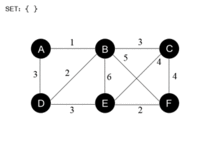This animation shows how the Prim's algorithm runs in a graph.(Click the image to see the animation)

## Proof of correctness

Let P be a connected, weighted graph. At every iteration of Prim's algorithm, an edge must be found that connects a vertex in a subgraph to a vertex outside the subgraph. Since P is connected, there will always be a path to every vertex. The output Y of Prim's algorithm is a tree, because the edge and vertex added to Y are connected. Let Y1 be a minimum spanning tree of P. If Y1=Y then Y is a minimum spanning tree. Otherwise, let e be the first edge added during the construction of Y that is not in Y1, and V be the set of vertices connected by the edges added before e. Then one endpoint of e is in V and the other is not. Since Y1 is a spanning tree of P, there is a path in Y1 joining the two endpoints. As one travels along the path, one must encounter an edge f joining a vertex in V to one that is not in V. Now, at the iteration when e was added to Y, f could also have been added and it would be added instead of e if its weight was less than e. Since f was not added, we conclude that$w(f) \ge w(e).$

Let Y2 be the graph obtained by removing f from and adding e to Y1. It is easy to show that Y2 is connected, has the same number of edges as Y1, and the total weights of its edges is not larger than that of Y1, therefore it is also a minimum spanning tree of P and it contains e and all the edges added before it during the construction of V. Repeat the steps above and we will eventually obtain a minimum spanning tree of P that is identical to Y. This shows Y is a minimum spanning tree.

Wikimedia Foundation. 2010.

### Look at other dictionaries:

• Prim — may refer to either of the following:* Dolní Přím, a village in Bohemia, as Nieder Prim (Lower Prim) site of the Battle of Königgrätz * Prim, Arkansas in Cleburne County, Arkansas * a river in Baden Württemberg, see *Primitive (geometry), the… …   Wikipedia

• Prim-Dijkstra-Algorithmus — Der Algorithmus von Prim dient der Berechnung eines minimalen Spannbaumes in einem zusammenhängenden, ungerichteten, kantengewichteten Graphen. Der Algorithmus wurde 1930 von dem tschechischen Mathematiker Vojtěch Jarník entwickelt. 1957 wurde er …   Deutsch Wikipedia

• Algorithm — Flow chart of an algorithm (Euclid s algorithm) for calculating the greatest common divisor (g.c.d.) of two numbers a and b in locations named A and B. The algorithm proceeds by successive subtractions in two loops: IF the test B ≤ A yields yes… …   Wikipedia

• Maze generation algorithm — Maze generation algorithms are automated methods for the creation of mazes. This maze generated by modified version of Prim s algorithm, below. Contents 1 Graph theory based methods …   Wikipedia

• Algoritmo de Prim — El algoritmo de Prim es un algoritmo perteneciente a la teoría de los grafos para encontrar un árbol recubridor mínimo en un grafo conexo, no dirigido y cuyas aristas están etiquetadas. En otras palabras, el algoritmo encuentra un subconjunto de… …   Wikipedia Español

• Robert C. Prim — Robert Clay Prim (born 1921 in Sweetwater, Texas) is an American mathematician and computer scientist.In 1941, Prim received his B.S. in Electrical Engineering from Princeton University. Later in 1949, he received his Ph.D. in Mathematics there… …   Wikipedia

• Dijkstra's algorithm — Not to be confused with Dykstra s projection algorithm. Dijkstra s algorithm Dijkstra s algorithm runtime Class Search algorithm Data structure Graph Worst case performance …   Wikipedia

• Kruskal's algorithm — is an algorithm in graph theory that finds a minimum spanning tree for a connected weighted graph. This means it finds a subset of the edges that forms a tree that includes every vertex, where the total weight of all the edges in the tree is… …   Wikipedia

• Borůvka's algorithm — is an algorithm for finding a minimum spanning tree in a graph for which all edge weights are distinct.It was first published in 1926 by Otakar Borůvka as a method of constructing an efficient electricity network for Moravia. [cite journal | last …   Wikipedia

• Nearest-neighbor chain algorithm — In the theory of cluster analysis, the nearest neighbor chain algorithm is a method that can be used to perform several types of agglomerative hierarchical clustering, using an amount of memory that is linear in the number of points to be… …   Wikipedia